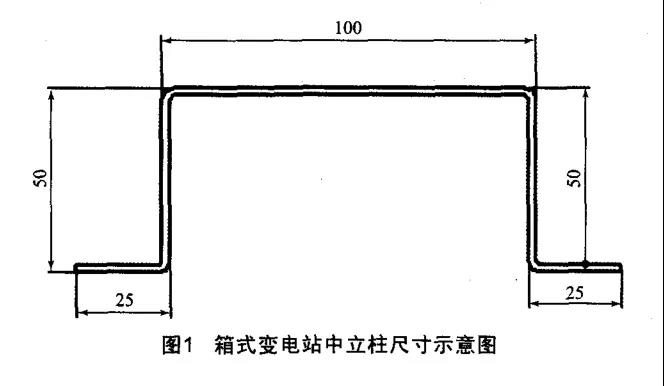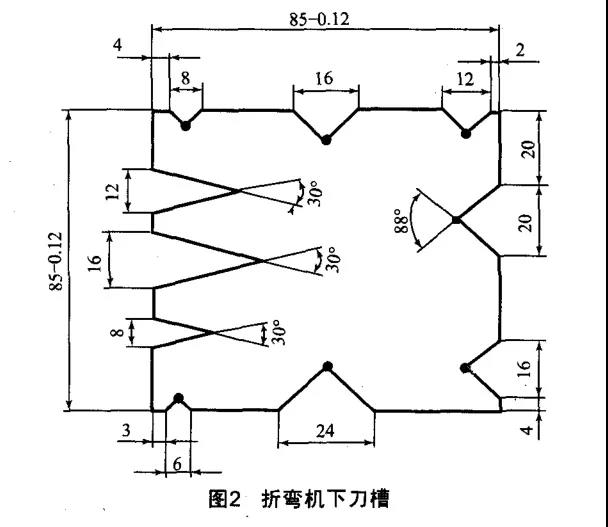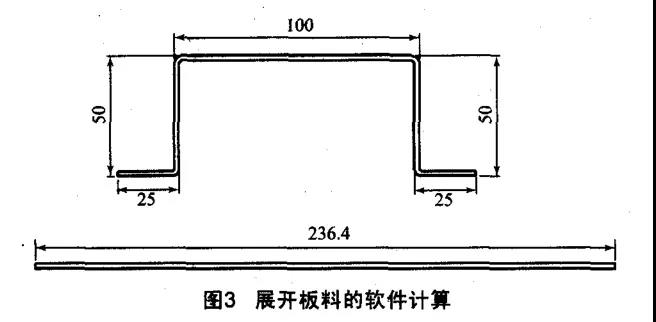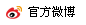# 【MFC推荐】钣金件折弯系数探讨

2019-11-04 15:16:34 来源： 网络C＝-0.075V+0.72T-0.01

C=-0.075 × 16+ 0.72 ×2 -0.01=0.23mm

L = L1+L2+L3+ ⋯  +Ln-2 × (n-1) ×T+(n-1) ×C

L=100+50+50+25+25-2×(5-1)X2+(5-1)×0.23=234.92mm

L= 100+50+50+25+25-4 X 1.75 × 2= 236 mm• 最新评论
暂无评论

### 技术文库